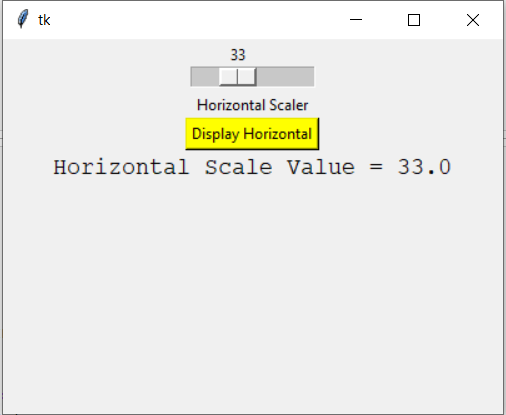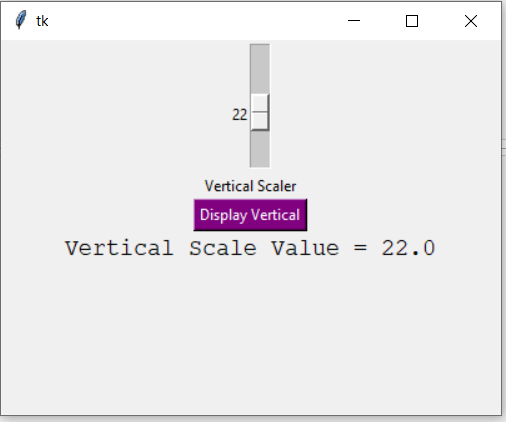# Python Tkinter – Scale Widget

• Difficulty Level : Medium
• Last Updated : 23 Dec, 2021

Tkinter is a GUI toolkit used in python to make user-friendly GUIs.Tkinter is the most commonly used and the most basic GUI framework available in python. Tkinter uses an object-oriented approach to make GUIs.

Note: For more information, refer to Python GUI – tkinter

## Scale widget

The Scale widget is used whenever we want to select a specific value from a range of values. It provides a sliding bar through which we can select the values by sliding from left to right or top to bottom depending upon the orientation of our sliding bar.

Syntax:

```S = Scale(root, bg, fg, bd, command, orient, from_, to, ..)
```

Optional parameters

• root – root window.
• bg – background colour
• fg – foreground colour
• bd – border
• orient – orientation(vertical or horizontal)
• from_ – starting value
• to – ending value
• troughcolor – set colour for trough.
• state – decides if the widget will be responsive or unresponsive.
• sliderlength – decides the length of the slider.
• label – to display label in the widget.
• highlightbackground – the colour of the focus when widget is not focused.
• cursor – The cursor on the widget which could be arrow, circle, dot etc.

Methods

• set(value) – set the value for scale.
• get() – get the value of scale.

Example 1: Creating a horizontal bar

 `# Python program to demonstrate``# scale widget`` ` `from` `tkinter ``import` `*` ` ` ` ` `root ``=` `Tk()  ``root.geometry(``"400x300"``) `` ` `v1 ``=` `DoubleVar()`` ` `def` `show1():  ``     ` `    ``sel ``=` `"Horizontal Scale Value = "` `+` `str``(v1.get())``    ``l1.config(text ``=` `sel, font ``=``(``"Courier"``, ``14``))  `` ` ` ` `s1 ``=` `Scale( root, variable ``=` `v1, ``           ``from_ ``=` `1``, to ``=` `100``, ``           ``orient ``=` `HORIZONTAL)   `` ` `l3 ``=` `Label(root, text ``=` `"Horizontal Scaler"``)`` ` `b1 ``=` `Button(root, text ``=``"Display Horizontal"``, ``            ``command ``=` `show1, ``            ``bg ``=` `"yellow"``)  `` ` `l1 ``=` `Label(root)`` ` ` ` `s1.pack(anchor ``=` `CENTER) ``l3.pack()``b1.pack(anchor ``=` `CENTER)``l1.pack() `` ` `root.mainloop()`

Output:Example 2: Creating a vertical slider

 `from` `tkinter ``import` `*`` ` `root ``=` `Tk()  ``root.geometry(``"400x300"``) ``v2 ``=` `DoubleVar()`` ` `def` `show2():``     ` `    ``sel ``=` `"Vertical Scale Value = "` `+` `str``(v2.get()) ``    ``l2.config(text ``=` `sel, font ``=``(``"Courier"``, ``14``))`` ` `s2 ``=` `Scale( root, variable ``=` `v2,``           ``from_ ``=` `50``, to ``=` `1``,``           ``orient ``=` `VERTICAL) `` ` `l4 ``=` `Label(root, text ``=` `"Vertical Scaler"``)`` ` `b2 ``=` `Button(root, text ``=``"Display Vertical"``,``            ``command ``=` `show2,``            ``bg ``=` `"purple"``, ``            ``fg ``=` `"white"``)`` ` `l2 ``=` `Label(root)`` ` `s2.pack(anchor ``=` `CENTER) ``l4.pack()``b2.pack()``l2.pack()`` ` `root.mainloop()`

Output:My Personal Notes arrow_drop_up